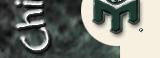# Conversions

All the terms and measures employed in "Cheap Eats" are standard American. For those of you who might be accessing "Cheap Eats" from places where these terms and measures are not used, here are some explanations and conversions.

## Conversions

Volume

• 1 tsp. = 5 ml
• 1 Tbs. = 15 ml
• 2 Tbs. = 30 ml or 1 fl. oz.
• 1 cup = 240 ml or 8 fl. oz. (vs. 10 oz. per cup in Imperial measure)
• 1 pt = 480 ml or 16 fl. oz.
• 1 qt = 960 ml or 32 fl. oz.

Weight

• 1 oz. = 28 gm
• 1 lb. = 454 gm
• 2.2 lb. = 1 kg

Length

• 1 in. = 2.54 cm.

Temperature

• 32°F = 0°C (water freezes)
• 212°F = 100°C (water boils)

Conversion formula:

• °C = 5/9(°F - 32)
e.g., 400°F is approximately 200°C

## Abbreviations

• tsp. = teaspoon
• Tbs. = tablespoon
• lb. = pound
• oz. = ounce (weight)
• fl. oz. = fluid ounce (volume)
• pt = pint
• qt = quart
• in. = inch
• ml = milliliter
• gm = gram
• cm. = centimeter
• F = Fahrenheit